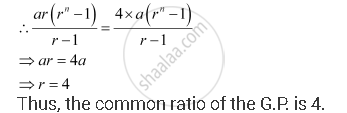CBSE (Arts) Class 11CBSE
Share
Notifications

View all notifications
Books Shortlist
Your shortlist is empty

# A G.P. Consists of an Even Number of Terms. If the Sum of All the Terms is 5 Times the Sum of Terms Occupying Odd Places, Then Find Its Common Ratio. - CBSE (Arts) Class 11 - Mathematics

Login
Create free account

Forgot password?
ConceptGeometric Progression (G. P.)

#### Question

A G.P. consists of an even number of terms. If the sum of all the terms is 5 times the sum of terms occupying odd places, then find its common ratio.

#### Solution

Let the G.P. be T1, T2, T3, T4, … T2n.

Number of terms = 2n

According to the given condition,

T1 + T2 + T3 + …+ T2n = 5 [T1 + T3 + … +T2n–1]

⇒ T1 + T2 + T3 + … + T2n – 5 [T1 + T3 + … + T2n–1] = 0

⇒ T2 + T4 + … + T2n = 4 [T1 + T3 + … + T2n–1]

Let the G.P. be aarar2ar3, …Is there an error in this question or solution?

#### APPEARS IN

NCERT Solution for Mathematics Textbook for Class 11 (2018 to Current)
Chapter 9: Sequences and Series
Q: 11 | Page no. 199
Solution A G.P. Consists of an Even Number of Terms. If the Sum of All the Terms is 5 Times the Sum of Terms Occupying Odd Places, Then Find Its Common Ratio. Concept: Geometric Progression (G. P.).
S